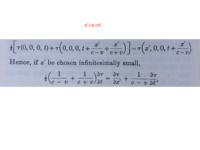# cannot figure out an equation in special relativity

#### bll230

##### New member
I am reading Einstein's original paper and trying to understand his work. I am an engineer, not a physicist, and the math in special relativity is not overly complicated, but I cannot duplicate one of his steps. He does leave out all the steps in his work.

This should be a simple differential of a parametric function, but I am missing something. My advanced calculus was 30 years ago, but I think (thought) I still have a reasonable grasp. A function tau of x' and t, where x' is itself a function of t.

Thanks

JohnLast edited:

#### firemath

##### Full Member
Hrm.....I think that none shall pass. This thread has been unanswered for a while now.

#### Cubist

##### Junior Member
For small b, then...

f(a+b) ≃ f(a) + f'(a)*b

This approximation works because it assumes f is linear for a small distance around "a".

Use this on the first line from your image. For the RHS its a little more tricky because there are two params of interest with deltas. I think if f(x,y) has partial derivatives df/dx and df/dy then f(x+b,y+c) ≃ f(x,y) + df/dx(x,y) * b + df/dy(x,y) * c , for small b and c.

You'll find that the same term "τ(0,0,0,t)" appears (added) on both LHS and RHS, so this can be removed. And then you can divide through by common factor x'. I'll leave the detail to you (since I'm only 85% sure that this'll work). Good luck

•firemath